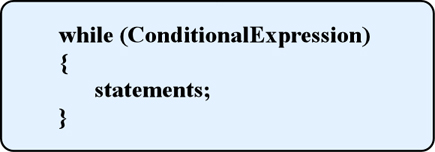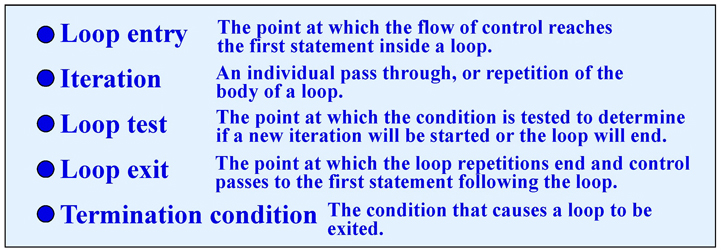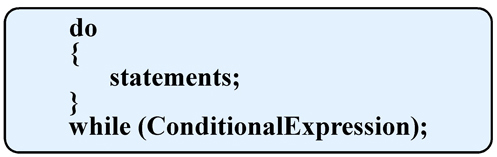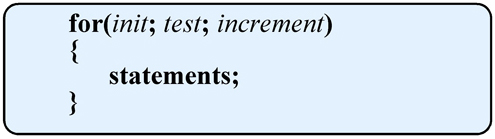# Loops

A Loop is a control structure that causes a statement or group of statements to be executed repeatedly. Frequently in a program you will want to repeat an action several times or until some condition is met. We do this in a loop. For example:
• To find the sum of a list of numbers you use a loop to repeatedly get the next value in the list and add it to a running total.
• If your program is monitoring a temperature reading you may repeatedly input a reading until you reach a certain temperature.
• If you are playing a guess a number game you want the user to repeatedly enter a guess until they guess the correct number.
• If you are playing an adventure game you want the user to repeatedly enter a command until they reach a goal or choose to quit playing.
In your programs you will use three types of loops.Which type you use will be determined by what you are trying to do, the conditions of the loop, and what will determine when the loop should terminate. The three types of loops in C/C++ are while, do-while, and for. Each one is covered below.

# The while LoopBasic layout of the while loop:The conditional expression used here is the same type of conditional expression used in the if command. An if statement is used to choose a course of action. A while statement is used to repeat some action as long as the conditional expression is true.

### Terms Related to Loops### The Count-Controlled while loop

In this while loop an action is repeated a given number of times. A counter variable is created and initialized to a starting value before the loop is started. The condition that is tested before each iteration of the loop is whether the counter has reached a predetermined value. The counter itself is incremented inside the while loop. In this example the counter, loopCount, is initialized to 1 and incremented in the loop until its' value is 10. You could also initialize loopCount to 10 then each time through the loop decrement it (loopCount--) testing (loopCount > 0).
```	// Count controlled loop
#include<iostream>
using namespace std;

int main()
{
int loopCount;		// loop control variable
loopCount = 1;
while(loopCount <= 10)
{
cout << "Hello! Loop count = " << loopCount << endl;
loopCount++;	// Increment control variable
}
return 0;
}
```

### The Event-Controlled while loop

In this while loop an action is repeated until a certain event occurs. This is by far the most frequently used form of the while loop. There are three different types of Event-Controlled while loops.
1. Sentinel-controlled loops - A sentinel variable is initialized to a specific value. The while loop continues until, through some action inside the loop, the sentinel variable is set to a predefined termination value. In the following example the user is asked to type characters at the keyboard, which are then echoed to the screen, then press the Enter key when done. Pressing the Enter key sets the char variable inChar to the newline character (defined by the special characer '\n').
```
// Sentinel-controlled loop
#include<iostream>
using namespace std;

int main()
{
char inChar;			// Input variable
cin.get(inChar);		// Init sentinel variable
while(inChar != '\n')
{
cout << inChar;		// Print the character
cin.get(inChar);	// Get the next character
}
cout << endl;
return 0;
}
```
2. End-of-file controlled loops - This type of while loop is usually used when reading from a file. The loop terminates when the end of the file is detected. This can easily be determined by checking the EOF() function after each read from the file. This function will return true when the end-of-file is reached. In the sample code below integer values are read from a data file until the end of the file is reached.
```	// EOF controlled loop
#include<iostream>
#include<fstream>
using namespace std;

int main()
{
ifstream   inData;
int        intValue;
inData.open("myData.txt");
if(!inData.good()
{
cout << "Failed to open myData.txt. Program terminating.\n";
return 0
}
while(!inData.EOF())
{
inData >> intValue;
cout << "Got value: " << intValue << endl;
}
inData.close();
return 0;
}
```
3. Flag controlled loops - A bool variable is defined and initialized to serve as a flag. The while loop continues until the flag variable value flips (true becomes false or false becomes true). In the following example the user is asked to enter numbers at the keyboard which are added together. To stop the addition the user enters a negative number.
```	// Flag controlled loop
#include<iostream>
using namespace std;

int main()
{
double sum = 0.0;
double value;
bool nonNegative = true;	// Init flag
while(nonNegative)		// Test if nonNegative is true
{
cin >> value;
if(value < 0)
nonNegative = false;
else
sum += value;
}
cout << "Sum of input numbers is " << sum << endl;
return 0;
}
```

### Designing a loop

It take programming skill to design a good loop. There are sevral questions you should answer to guide you in this process.

Questions which should be asked when designing any loop structure
1. What is the condition that ends the loop?
2. How should the condition be initialized?
3. How should the condition be updated?
4. What is the process being repeated?
5. How should the process be initialized?
6. How should the process be updated?
7. What is the state of the program on exiting the loop?

### Nested loops

You can, and quite frequently will need to, nest loops inside of other loops. Here is a simple example:
```	// Nested loops
#include<iostream>
using namespace std;

int main()
{
int limit1 = 3;		// Set number of times to execute outer loop
int limit2 = 5;		// Set number of times to execute inner loop
int outCount = 0;	// Create and init outer loop counter
int inCount;		// Create inner loop counter
while(outCount < limit1)
{
inCount = 0;	// Init inner loop counter
while(inCount < limit2)
{
cout << "outCount = " << outCount << "  inCount = " << inCount << endl;
}
}
return 0;
}
```

# The do-while LoopBasic layout of the do-while loop:In the while loop we have to be careful to properly initialize the condition being testing in the loop. If that condition is false at the start then nothing inside the loop is ever executed. Sometimes we want to make sure we execute the loop at least once. The do-while loop is just right for this. In a do-while loop the condition is not tested until the end of an iteration through the loop, therefore it will always execute at least one. Notice it is in essence a while loop turned upside down. Here is a simple example of a do-while loop:
```	// Count controlled loop
#include<iostream>
using namespace std;

int main()
{
int loopCount;		// loop control variable
loopCount = 1;
do
{
cout << "Hello! Loop count = " << loopCount << endl;
loopCount++;	// Increment control variable
}
while(loopCount <= 10);
return 0;
}
```

# The for LoopBasic layout of the for loop:The while and do-while loops are meant to be used when you do not know how many times you will need to repeat an action. Some condition will change in the loop to terminate it. If you do know exactly how many iterations you will be making then the for loop is the better choice. There are three parts inside the parentheses of a for loop statement. The first is where the loop counter variable is initialized to a starting value. You can declare and initialize the variable here or just use a previously declared integer variable. The second part of the for statement is the test which determines when the loop terminates. The third part is the increment. This can be a simple increment by 1 (loopCount++ or loopCount += 1 or loopCount = loopCount +1), or an increment by any other amount (loopCount += 2 or loopCount = loopCount + 2). You can also initialize the loop counter variable to a larger number, test while the loop counter is greater than zero, and then use a decrement of the loop counter value [for(int i=10; i>0; i--)]. BTW there is a long tradition of using variables named i, j, or k as loop counter variables because these were reserved variable names in the Fortran programming language back in the 1950s and 1960s.

Below is a sample of a for loop. Note that this is the same count-controlled loop that is shown above using both a while and a do-while loop. In this example the loop counter variable, loopCount, is declared inside the for loop statement. Once the for loop exits this variable will ceast to exist. If you want to use the variable again you will need to declare it above the for statement and then only initialize it (loopCount=1) in the for statement.
```	// Count controlled loop
#include<iostream>
using namespace std;

int main()
{
for(int loopCount=1; loopCount<=10; loopCount++)
{
cout << "Hello! Loop count = " << loopCount << endl;
loopCount++;	// Increment control variable
}
return 0;
}
```

### Designing a loop

It take programming skill to design a good loop. There are sevral questions you should answer to guide you in this process.

Questions which should be asked when designing any loop structure
1. What is the condition that ends the loop? In most cases this is stright forward, but make sure you know exactly what that condition is and make sure it can occur.
2. How should the condition be initialized? Make sure the loop control variable is appropriately initialized so the loop doesn't terminate immediate before doing anything. If you fail to properly initialize the pre-conditions before starting a loop the results can be undefined.
3. How should the condition be updated? Is this taken care of automatically by comparing input values to a sentinel value, or do you have to increment a counter, check a flag, etc.
4. What is the process being repeated? What action is coded inside the loop to be repeated?
5. How should the process be initialized? Are there any variables, etc. that need to be initialized in addition to the loop control variable before starting the loop? For example, if summing a list of values you need to initialize sum to zero. This is not done automatically when you create the variable.
6. How should the process be updated? What processes are executed inside the loop? For example, in summing a list of values: (1) get the next value, (2) add it to the running sum.
7. What is the state of the program on exiting the loop? If there anything you need to do to clean up, like closing files that were used in the loop, or outputting values calculated in the loop?